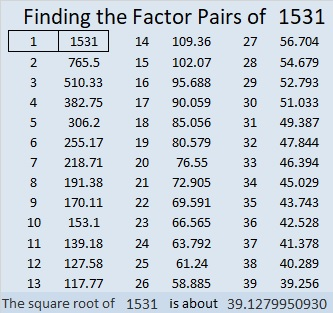# 1531 Spider’s Web or Not?

Contents

### Today’s Puzzle:

Even though1531 is a prime number, what geometric shape can you arrange 1531 tiny squares into?

As you look at the graphic below, ask yourself a couple of questions. What do you notice? What do you wonder?You might think that you are looking at a spider’s web with the numbers 1, 11, 31, 61, 101, 151, 211, 281, 361, 451, 551, 661, 781, 911, 1051, 1201, 1361, and 1531 trapped inside.

All of those numbers are centered decagonal numbers. My puzzle for you today is: If it were a spider’s web, and a spider ate the last digit of each of those centered decagonal numbers, what kind of figurate number would be left behind in every case?

### Factors of 1531:

• 1531 is a prime number.
• Prime factorization: 1531 is prime.
• 1531 has no exponents greater than 1 in its prime factorization, so √1531 cannot be simplified.
• The exponent in the prime factorization is 1. Adding one to that exponent we get (1 + 1) = 2. Therefore 1531 has exactly 2 factors.
• The factors of 1531 are outlined with their factor pair partners in the graphic below.

How do we know that 1531 is a prime number? If 1531 were not a prime number, then it would be divisible by at least one prime number less than or equal to √1531. Since 1531 cannot be divided evenly by 2, 3, 5, 7, 11, 13, 17, 19, 23, 29, 31, or 37, we know that 1531 is a prime number.### Another Fact about the Number 1531:

OEIS.org informs us that 1531 = 5494153169521.

This site uses Akismet to reduce spam. Learn how your comment data is processed.